Published © CC BY

# Autonomous (LIDAR) Litter Detection Robot w/ Edge Impulse

Recognize and monitor litter with object detection via this self-driving robot. It also deploys a video stream and a fall detection system.

ExpertFull instructions provided6 hours8,467

## Things used in this project

### Hardware components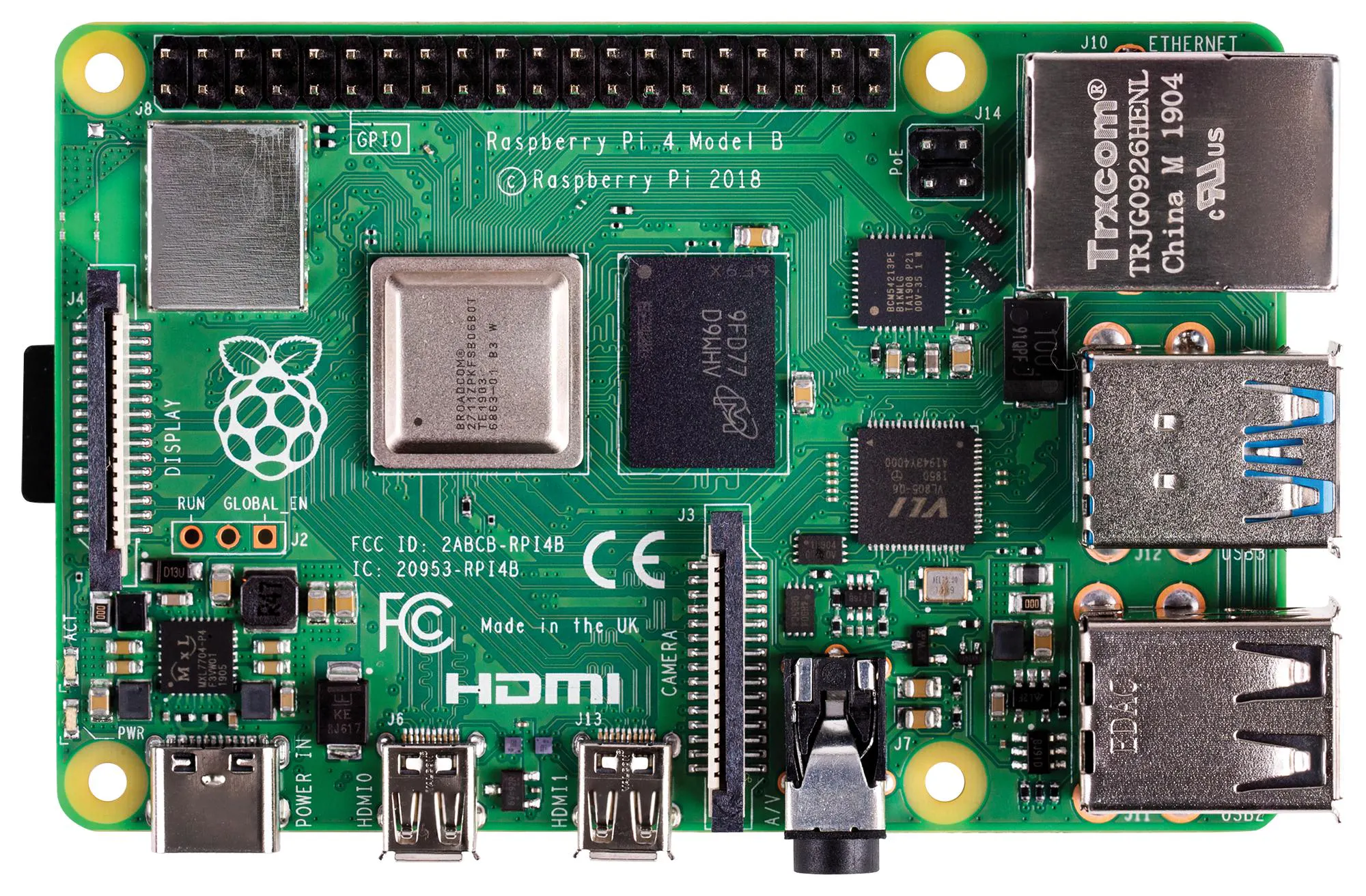Raspberry Pi 4 Model B
×1
 DFRobot RPLIDAR A1M8-R6 - 360 Degree Laser Scanner
×1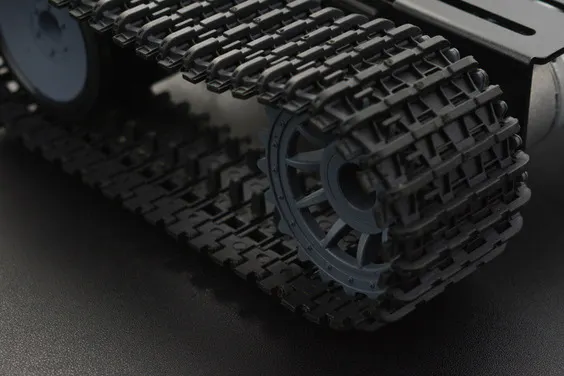DFRobot Black Gladiator - Tracked Robot Chassis
×1
 USB Webcam
×1
 L298N Motor Driver Module
×1Arduino Nano R3
×1
 DFRobot Serial 6-Axis Accelerometer
×1
 SSD1306 OLED 128x32
×1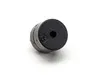Buzzer
×1
 USB Buck-Boost Converter Board
×1
 DFRobot 8.9' 1920x1200 IPS Touch Display
×1
 Xiaomi 20000 mAh 3 Pro Type-C Power Bank
×1
 Male-Female Brass Hex PCB Spacer Standoff
×20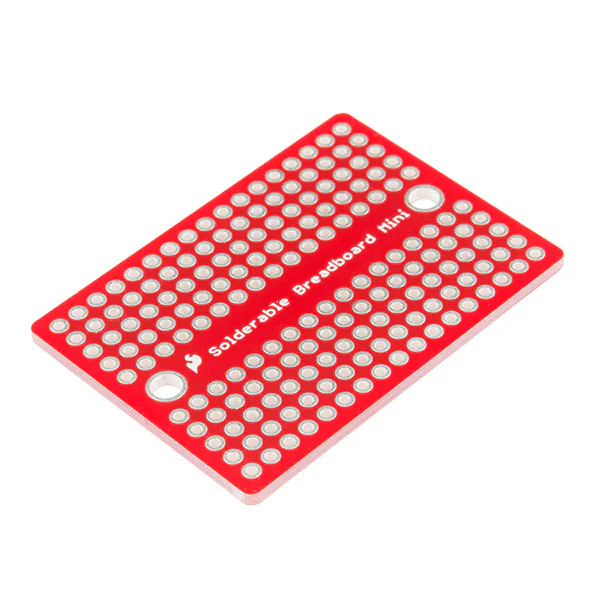SparkFun Solder-able Breadboard - Mini
×2Jumper wires (generic)
×1

### Software apps and online servicesEdge Impulse Studio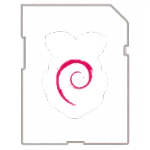Raspberry Pi Raspbian
 ThonnyArduino IDE

### Hand tools and fabrication machinesHot glue gun (generic)

## Schematics

### Connections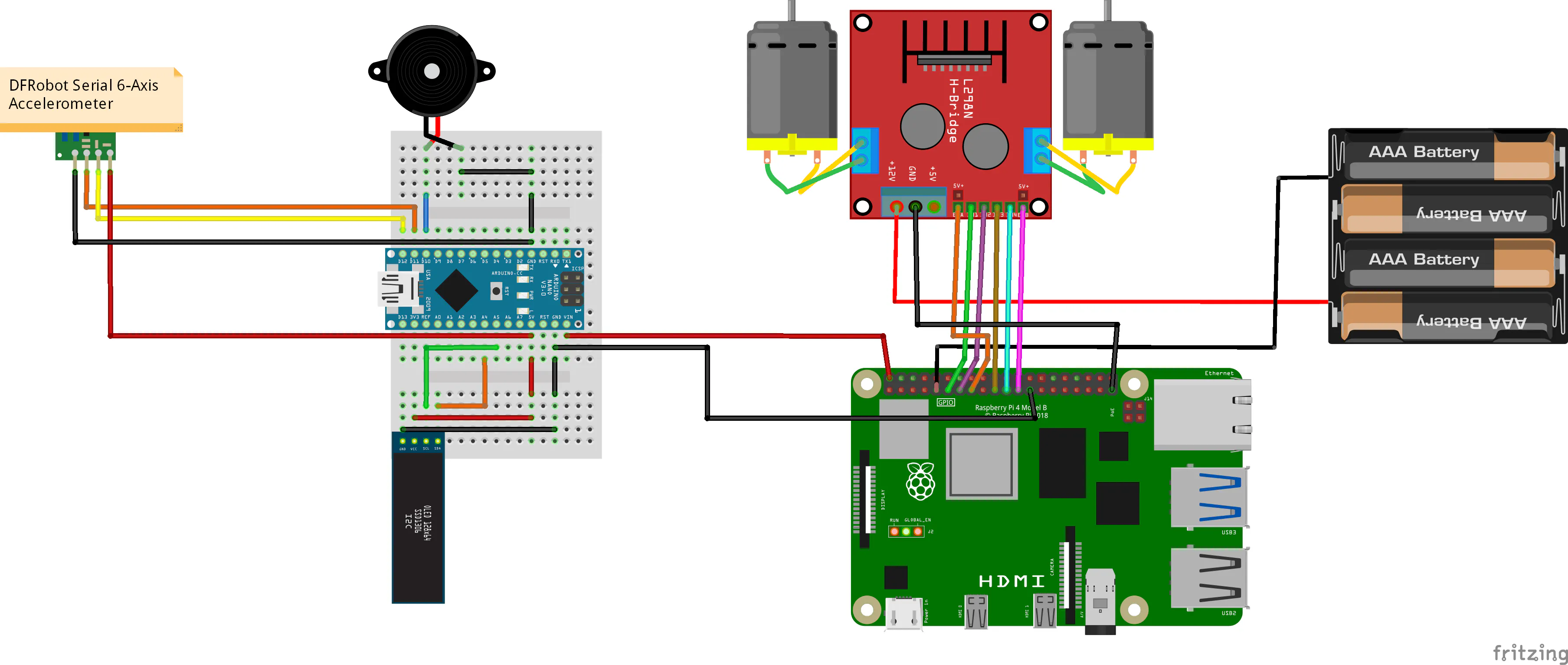## Code

### LIDAR_Litter_Detection_Robot.py

Python
```# LIDAR Litter Detection Robot w/ Edge Impulse

# Raspberry Pi 4

# By Kutluhan Aktar

# Recognize and monitor litter with object detection via this self-driving robot.
# It also deploys a video stream and a fall detection system.

# https://www.theamplituhedron.com/projects/Autonomous_(LIDAR)_Litter_Detection_Robot_w_Edge_Impulse/

from adafruit_rplidar import RPLidar
from math import floor
import RPi.GPIO as GPIO
from time import sleep

# Create the Litter Detection Robot class with the required settings:
class Litter_Detection_Robot:
def __init__(self, mot_pin_1, mot_pin_2, mot_pin_3, mot_pin_4, mot_pin_5, mot_pin_6):
# Setup the RPLidar:
PORT_NAME = '/dev/ttyUSB0'
self.lidar = RPLidar(None, PORT_NAME, timeout=30)
self.con_scan = True
# Define the L298N motor driver board pins:
self.in_1_1 = mot_pin_1
self.in_1_2 = mot_pin_2
self.en_1 = mot_pin_3
self.in_2_1 = mot_pin_4
self.in_2_2 = mot_pin_5
self.en_2 = mot_pin_6
# Set GPIO mode:
GPIO.setmode(GPIO.BCM)
# Get information from the RPLidar:
def get_data_from_LIDAR(self):
# Record the data generated by the RPLidar.
if(self.con_scan):
try:
print(self.lidar.info)
for scan in self.lidar.iter_scans():
self.scan_data = *360
for (_, angle, distance) in scan:
self.scan_data[min([359, floor(angle)])] = distance
self.process_lidar_data(60, 120, 240, 300, 340, 20)
except KeyboardInterrupt:
self.con_scan = False
print("\nLIDAR Stopped!")
self.lidar.stop()
self.lidar.disconnect()
GPIO.cleanup()
# Process the data generated by the RPLidar to control the robot chassis.
def process_lidar_data(self, r_ang_s, r_ang_e, l_ang_s, l_ang_e, f_ang_s, f_ang_e, t=400, _range=16):
# Object Distance:
r_start = r_end = l_start = l_end = f_start = f_end = 3000
# Debugging the data generated by the RPLidar:
for i in range(_range):
_angle = r_ang_s + i
if(self.scan_data[_angle] > 1):
r_start = self.scan_data[_angle]
#print("Right Dis. Start = " + str(r_start))
break
for i in range(_range):
_angle = r_ang_e - i
if(self.scan_data[_angle] > 1):
r_end = self.scan_data[_angle]
#print("Right Dis. End = " + str(r_end))
break

for i in range(_range):
_angle = l_ang_s + i
if(self.scan_data[_angle] > 1):
l_start = self.scan_data[_angle]
#print("Left Dis. Start = " + str(l_start))
break
for i in range(_range):
_angle = l_ang_e - i
if(self.scan_data[_angle] > 1):
l_end = self.scan_data[_angle]
#print("Left Dis. End = " + str(l_end))
break

for i in range(_range):
_angle = f_ang_s + i
if(self.scan_data[_angle] > 1):
f_start = self.scan_data[_angle]
#print("Front Dis. Start = " + str(f_start))
break
for i in range(_range):
_angle = f_ang_e - i
if(self.scan_data[_angle] > 1):
f_end = self.scan_data[_angle]
#print("Front Dis. End = " + str(f_end))
break

# Control the robot chassis according to the debugged distance values:
if((r_start < t) and (r_end < t)):
print("\nStop!")
self.robot_chassis_controls("stop")
sleep(0.5)
print("Right!")
self.robot_chassis_controls("left", speed="moderate")
sleep(1)
print("Go!")
self.robot_chassis_controls("forward", speed="low")
if((l_start < t) and (l_end < t)):
print("\nStop!")
self.robot_chassis_controls("stop")
sleep(0.5)
print("Left!")
self.robot_chassis_controls("right", speed="moderate")
sleep(1)
print("Go!")
self.robot_chassis_controls("forward", speed="low")
if((f_start < t) and (f_end < t)):
print("\nStop!")
self.robot_chassis_controls("stop")
sleep(0.5)
print("Front!")
self.robot_chassis_controls("turn", speed="moderate")
sleep(1)
print("Go!")
self.robot_chassis_controls("forward", speed="low")

# Initiate the robot chassis:
def robot_chassis_init(self):
# Define the motor driver pin settings:
GPIO.setup(self.in_1_1, GPIO.OUT)
GPIO.setup(self.in_1_2, GPIO.OUT)
GPIO.setup(self.en_1, GPIO.OUT)
GPIO.setup(self.in_2_1, GPIO.OUT)
GPIO.setup(self.in_2_2, GPIO.OUT)
GPIO.setup(self.en_2, GPIO.OUT)
# Default:
GPIO.output(self.in_1_1, GPIO.HIGH)
GPIO.output(self.in_1_2, GPIO.LOW)
GPIO.output(self.in_2_1, GPIO.HIGH)
GPIO.output(self.in_2_2, GPIO.LOW)
self.s_1 = GPIO.PWM(self.en_1, 100)
self.s_1.start(25)
self.s_2 = GPIO.PWM(self.en_2, 100)
self.s_2.start(25)
# Control the robot chassis:
def robot_chassis_controls(self, command, speed="default"):
# Directions:
if(command == "forward"):
GPIO.output(self.in_1_1, GPIO.HIGH)
GPIO.output(self.in_1_2, GPIO.LOW)
GPIO.output(self.in_2_1, GPIO.HIGH)
GPIO.output(self.in_2_2, GPIO.LOW)
elif(command == "left"):
GPIO.output(self.in_1_1, GPIO.LOW)
GPIO.output(self.in_1_2, GPIO.LOW)
GPIO.output(self.in_2_1, GPIO.HIGH)
GPIO.output(self.in_2_2, GPIO.LOW)
elif(command == "right"):
GPIO.output(self.in_1_1, GPIO.HIGH)
GPIO.output(self.in_1_2, GPIO.LOW)
GPIO.output(self.in_2_1, GPIO.LOW)
GPIO.output(self.in_2_2, GPIO.LOW)
elif(command == "turn"):
GPIO.output(self.in_1_1, GPIO.LOW)
GPIO.output(self.in_1_2, GPIO.HIGH)
GPIO.output(self.in_2_1, GPIO.HIGH)
GPIO.output(self.in_2_2, GPIO.LOW)
elif(command == "stop"):
GPIO.output(self.in_1_1, GPIO.LOW)
GPIO.output(self.in_1_2, GPIO.LOW)
GPIO.output(self.in_2_1, GPIO.LOW)
GPIO.output(self.in_2_2, GPIO.LOW)
# Speed:
if(speed == "low"):
self.s_1.ChangeDutyCycle(25)
self.s_2.ChangeDutyCycle(25)
elif(speed == "normal"):
self.s_1.ChangeDutyCycle(50)
self.s_2.ChangeDutyCycle(50)
elif(speed == "moderate"):
self.s_1.ChangeDutyCycle(75)
self.s_2.ChangeDutyCycle(75)
elif(speed == "high"):
self.s_1.ChangeDutyCycle(90)
self.s_2.ChangeDutyCycle(90)

# Define a new class object named 'robot':
robot = Litter_Detection_Robot(17, 27, 22, 10, 9, 11)
robot.robot_chassis_init()

while True:
robot.get_data_from_LIDAR()
```

### lidar_litter_detection_robot_accelerometer.ino

Arduino
```         /////////////////////////////////////////////
//      LIDAR Litter Detection Robot       //
//             w/ Edge Impulse             //
//             ---------------             //
//              (Arduino Nano)             //
//             by Kutluhan Aktar           //
//                                         //
/////////////////////////////////////////////

//
// Recognize and monitor litter with object detection via this self-driving robot. It also deploys a video stream and a fall detection system.
//
// https://www.theamplituhedron.com/projects/Autonomous_(LIDAR)_Litter_Detection_Robot_w_Edge_Impulse/
//
//
// Connections
// Arduino Nano :
//                                DFRobot Serial 6-Axis Accelerometer
// D11 --------------------------- TX
// D12 --------------------------- RX
//                                SSD1306 OLED 128x32
// A4  --------------------------- SDA
// A5  --------------------------- SCL
//                                Buzzer
// D10 --------------------------- S

// Include the required libraries.
#include <DFRobot_WT61PC.h>
#include <SoftwareSerial.h>

// Initiate the software serial port. RX11 TX12
SoftwareSerial mySerial(11, 12);

// Define the Serial 6-Axis Accelerometer.
DFRobot_WT61PC accelerometer(&mySerial);

// Define the SSD1306 screen settings:
#define SCREEN_WIDTH 128 // OLED display width, in pixels
#define SCREEN_HEIGHT 32 // OLED display height, in pixels
#define OLED_RESET    -1 // Reset pin # (or -1 if sharing Arduino reset pin)

Adafruit_SSD1306 display(SCREEN_WIDTH, SCREEN_HEIGHT, &Wire, OLED_RESET);

// Define monochrome graphics:
static const unsigned char PROGMEM _speed [] = {
0x00, 0x7E, 0x00, 0x01, 0xFF, 0x80, 0x07, 0xFF, 0xE0, 0x0F, 0x99, 0xF0, 0x1E, 0x18, 0x78, 0x3C,
0x18, 0x3C, 0x3E, 0x00, 0x7C, 0x76, 0x00, 0xEE, 0x72, 0x00, 0x0E, 0x60, 0x01, 0x06, 0xE0, 0x07,
0x07, 0xE0, 0x1E, 0x07, 0xE0, 0x3E, 0x07, 0xF8, 0x26, 0x1F, 0xF8, 0x3C, 0x1F, 0xC0, 0x18, 0x03,
0xE0, 0x00, 0x07, 0x60, 0x00, 0x06, 0x60, 0x00, 0x06, 0x73, 0x00, 0xCE, 0x3E, 0x00, 0x7C, 0x3C,
0x00, 0x7C, 0x1C, 0x00, 0x38, 0x08, 0x00, 0x10,
};

// Define the buzzer pin.
#define buzzer 10

void setup(){
mySerial.begin(9600);

// Revise the data output frequency of the accelerometer: FREQUENCY_0_1HZ for 0.1Hz, FREQUENCY_0_5HZ for 0.5Hz, FREQUENCY_1HZ for 1Hz, FREQUENCY_2HZ for 2Hz, FREQUENCY_5HZ for 5Hz, FREQUENCY_10HZ for 10Hz, FREQUENCY_20HZ for 20Hz, FREQUENCY_50HZ for 50Hz, FREQUENCY_100HZ for 100Hz, FREQUENCY_125HZ for 125Hz, FREQUENCY_200HZ for 200Hz.
accelerometer.modifyFrequency(FREQUENCY_10HZ);

// Initialize the SSD1306 screen:
display.begin(SSD1306_SWITCHCAPVCC, 0x3C);
display.display();
delay(1000);
}

void loop(){
if(accelerometer.available()){
// Print the acceleration information of the X, Y, and Z-axis.
display.clearDisplay();
display.drawBitmap(100, 6, _speed, 24, 24, SSD1306_WHITE);
display.setTextSize(1);
display.setCursor(0,0);
display.setTextColor(SSD1306_WHITE);
display.print("X: ");
display.print(accelerometer.Acc.X);
display.print(" Y: ");
display.println(accelerometer.Acc.Y);
display.print("\nZ: ");
display.print(accelerometer.Acc.Z);
display.display();

// Activate the buzzer depending on the angle information of the X, Y, Z-axis.
if(accelerometer.Angle.X < -50 || accelerometer.Angle.Y < -50 /*|| accelerometer.Angle.Z < -160*/){
tone(buzzer, 500);
delay(250);
}else{
noTone(buzzer);
}
}
}
```

## Credits

### Kutluhan Aktar

66 projects • 215 followers
Self-Taught Full-Stack Developer | @EdgeImpulse Ambassador | Maker | Physics Enthusiast